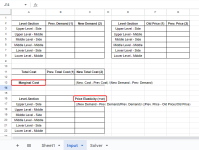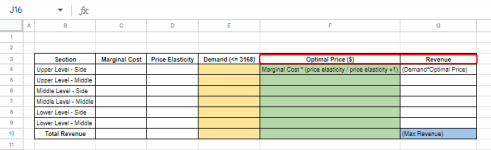# Ticket Pricing

#### wanderson88

##### New member
Good morning. I am attempting to solve a problem using solver that I built to complement a scenario that my students encountered while using a platform called knowledge matters. The long and short of it is that the students were tasked with figuring out the ticket prices for six sections in a stadium to generate \$575,000 in profit to pass the simulation. I am trying to figure out the optimal ticket pricing to maximize revenue instead of guessing and checking. The constraints that I have would be seating capacity with changing cells being the prices. The target cell is the total revenue. I have attached the sheet. Any assistance would be greatly appreciated.

Dynamic Ticket Pricing

•shamimarita
I am trying to figure out the optimal ticket pricing to maximize revenue instead of guessing and checking. The constraints that I have would be seating capacity with changing cells being the prices. The target cell is the total revenue.
Hello Wanderson,

Thank you for sharing the experience with us!

I understand you want to figure out the optimal ticket pricing to maximize revenue using the Solver tool. Fortunately, we came up with a way to do that. Here is the idea:

• In the Input sheet, populate the headers with the correct parameters as necessary.
• Now, calculate the Marginal Cost and Price Elasticity with the given formulas:

Marginal Cost= (New. Cost - Prev Cost) /(New Demand - Prev. Demand)

Price Elasticity= ((New Demand - Prev. Demand)/Prev. Demand)/ ((Prev. Price - Old Price)/Old Price)

• See the below screenshot for a better understanding.• Later, go to the Solver sheet >> find out the Optimal Price & Revenue with the following formulas:
Optimal Price= Marginal Cost * (price elasticity / price elasticity +1)

Revenue= (Demand*Optimal Price)

• Populate the other headers as needed.• Now, your data is ready for analysis. Open the Solver analysis tool >> input correct parameters >> Solve.

Note: The Max Revenue is your objective. The Constraints will be Demand in the yellow column. Lastly, Set the by changing values with the optimal prices in the green column.

If you need any help with the Solver tools, please follow the below article:

I have attached the result sheet here. Hope this helps! Thank you.

Ticket Pricing Analysis

Regards,
Yousuf Shovon

•shamimarita

Members online
0
Guests online
22
Total visitors
22